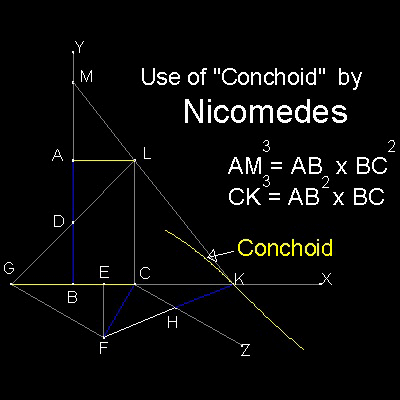Doubling the Cube
Go to   Fun_Math Content Table   Three Famous Problems   Delian Problem

### Doubling the cube by Greek Mathematicians

#### Conchoid by Nicomedes

Nicomedes invented a curve named "conchoid" to solve the Delian problem.
It is used to find a point K on X-axis such that line FK passes through
a point "H" on line CZ, and cuts x-axis while HK = CF is being satisfied.

So this process looks similar to "Verging"(neusis) used
by Archimedes, Pappus, and many Greek mathematicians in angle trisection.

#### Solution using Conchoid

 ``` Make rectangle ABCL. D,E are mid-points of AB and BC, Extend LD to produce G on x-axis. Erect EF perpendicular to x-axis and make CF = AD. Draw CZ parallel to GF. Choose a point K on x-axs such that HK = CF. Such a point is the intersection of special curve "conchoid" and x-axis. This curve can be drawn when F is "pole", CZ is "rule" and CF is "distance". Alternative is use a ruler marked with length CF on the edge, and find the location of FK (verging,or neusis process) Once point K is found, extend line KL to produce point M on y-axis. Then AM = {AB.BC2}1/3 and CK = {AB2.BC}1/3 ```You can see the process using "conchoid" in animation.
******** Nicomedes_Delian_desc.dwg *******

To create this drawing and animation:
Then from command line, type test_0

#### Solution using neusis(verging)

Alternative approach is the neusis (verging) technique used by Archimedes,Pappus and other Greek mathematicians in solving angle trisection problem.
A ruler marked with length CF is placed so that one marker is on line CZ and the other, on x-axis while this ruler passes through point F.
So this is an iterative process.

To create this drawing and animation:
Then from command line, type Nicomedes_Delian

#### Proof that AM and CK are the solutions

 ``` Heath gives the following proof: First step is to show MD = FK. Since E bisects BC, BK.KC + CE2 = KF2 (note: recall (a + b) (a - b) = a2 + b2 ) Add EF2 to both sides; BK.KC + CF2 = KF2 (1) Since AL is parallel to GK, so is CL to MB, MA / AB = ML / LK = BC / CK But AB = 2 AD, and BC = (1/2)GC Therefore MA / AD = GC /CK = FH / HK and MD / DA = FK / HK Here,DA = HK,therefore MD = FK Since D bisects AB, MD2 = BM.MA + DA2 Combining this with (1) because MD = FK, BM.MA + DA2 = BK.KC + CF2 But DA = CF; therefore BM.MA = BK.KC And CK/MA = BM/BK = LC/CK, Whereas, in triangles MAl & MBK, BM/BK = MA/AL Therefore LC/CK = CK/MA = MA/AL or AB/CK = CK/MA = MA/BC Then AM = {AB.BC2}1/3 and CK = {AB2.BC}1/3 ```

Go to   Fun_Math Content Table   Three Famous Problems   Delian Problem

All questions/suggestions should be sent to Takaya Iwamoto

Last Updated Nov 22, 2006